Online Tution   »   CBSE Class 12 Physics Term 2...

# CBSE Class 12 Physics Term 2 Additional Practice Questions 2022

## CBSE Class 12 Physics Term 2 Practice Questions 2022

CBSE Class 12 Physics Term 2 Practice Questions 2022 are important for CBSE Term 2 Exam 2022. The students must solve the CBSE Class 12 Physics Term 2 Practice Questions 2022 given on this page to get good marks in the physics subject. Physics is a subject that requires a proper understanding of concepts. The student can’t mug up physics concepts. The students appearing in CBSE Term 2 Exam 2022 must bookmark this page to get the latest updates from CBSE.

## CBSE Class 12 Physics Term 2 Practice Questions 2022

The Central Board of Secondary Education is all set to conduct CBSE Term 2 Exam 2022. The CBSE has released the date sheet of the CBSE Class 12 Term 2 Exam 2022 on its official website. As per the CBSE Class 12 Term 2 Datesheet, the Central Board of Secondary Education will conduct the Class 12 Term 2 examination of physics subject on the 20th of May 2022, Friday. Solve all the CBSE Class 12 Physics Term 2 Practice Questions 2022 here to assess your preparation for the physics subject.

## CBSE Class 12 Physics Term 2 Practice Questions 2022: Exam Pattern

We have provided the CBSE Term 2 Physics pattern as per the sample paper released by the Central Board of Secondary Education for Term 2. Preparing for any exam necessitates familiarity with the exam format. If required, students will be provided with a log table, and the use of calculators in exams will be restricted. The CBSE Term 2 Physics question paper is divided into three sections: Section A, Section B, and Section C. Check the further information provided below:

Section A: In this section, 3 questions of 2 marks each will be asked.

Section B: In this section, 8 questions of 3 marks each will be asked.

Section C: In this section, one case study-based question of 4 marks will be asked.

## CBSE Class 12 Physics Term 2 Practice Questions 2022

SECTION A
1. (a) An n-type semiconductor material is produced by adding impurity atoms to a pure semiconductor material. How many valence electrons will the impurity atoms have in their outer shell? What will the net charge be on the semiconductor material after adding the impurity atoms?

(b) What is the purpose of the following devices?
i. A photodiode in reverse bias

Ans. A photodiode operates in reverse bias because, as a photodetector or photosensor, it must conduct only when radiation strikes it. The dark current for zero illumination is negligibly small in the reverse biassed mode, on the order of a few picoamperes to nanoamperes.
ii. An LED in forward bias

Ans. Light-emitting diodes are built using a weak layer of heavily doped semiconductor material. Based on the semiconductor material used and the amount of doping, an LED will emit a colored light at a particular spectral wavelength when forward biased. Below is the demonstration of the LED symbol.

2. (a) An electron of mass m and a photon carry the same energy E. What is the ratio of de Broglie wavelength associated with the electron to the wavelength of the photon?

Ans.

(b) Why do macroscopic objects in our daily life not show wave-like properties whereas in the sub-atomic domain the wave-like character is significant?

Ans. They exhibit wave like nature but it is completely negligible matter for macroscopic bodies have very small wavelengths. According to De Broglie’s equation, wavelength is inversely proportional to mass of the object. As macroscopic bodies have have large mass when compared to micro objects, they cannot exhibit wave Nature.
OR
The table below lists the different transitions of an electron in a hydrogen atom: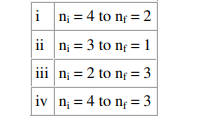Among these, identify:
(a) the transition that gives absorption spectra.
(b) the transition that gives Lyman spectral line, Balmer spectral line, and Brackett spectral line.

3. (a) Define barrier potential across a p-n junction. What is the impact on the barrier potential when the p-n junction is connected in forward bias?

Ans. A p-n junction is an interface or a boundary between two semiconductor material types, namely the p-type and the n-type, inside a semiconductor.

In forward biasing the positive terminal of the battery is connected to the the p-side and the negative terminal to the n-side of the p-n junction. The forward bias voltage opposes the potential barrier. Due to this, the potential barrier is reduced and hence the depletion layer becomes thin. The conduction across p-n junction takes place due to migration of majority carriers from one region to another. The resistance of p-n junction becomes low when forward biased.
(b) How does the current change as the voltage applied across the p-n junction varies from small to very high in reverse bias condition?

Ans. The flow of electrons from the n-side towards the p-side of the junction takes place when there is an increase in the voltage. Similarly, the flow of holes from the p-side towards the n-side of the junction takes place along with the increase in the voltage. This results in the concentration gradient between both sides of the terminals. Due to the formation of the concentration gradient, there will be a flow of charge carriers from higher concentration regions to lower concentration regions. The movement of charge carriers inside the pn junction is the reason behind the current flow in the circuit.

SECTION B

4. (a) A ray of light of wavelength λ falls normally on a right-angled isosceles prism ABC of refractive index n. Find the minimum value of refractive index n of the prism required for the total internal reflection of the light to occur on the face BC of the prism.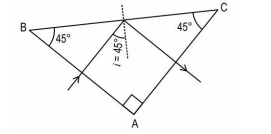(b) For any given thin prism of small angle A, refractive index n, and an incident blue light, answer the following questions.
i. Write the formula for angle of minimum deviation for a thin prism. If the whole arrangement is immersed in a liquid of refractive index n’ < n, how will the angle of minimum deviation change?
ii. For some angle of incidence on the second face of the prism, the incident blue light undergoes total internal reflection. However, a red incident light for the same angle of incidence on the second face of the prism does not undergo total internal reflection. Give reason.

OR
(a) A given glass slab WXYZ has a curved side on the left and a plane side on the right. On the left of the curved side, XPY is air and on the right side of the plane side, WZ is water. An object is present inside the glass slab at O beyond its center of curvature C.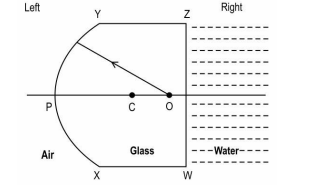Copy the diagram and complete the ray diagram to represent an image I as viewed from the left side. Also state whether this image is real or virtual.

(b) In a refracting type of telescope, what is the impact on its magnifying power if the objective and eyepiece lens are interchanged? Explain your answer.
(c) Give one advantage of using an objective lens with a large aperture in a telescope.

5. (a) What is the ratio of minimum to maximum energy of the radiations emitted by transition of an electron to the ground state of a hydrogen atom?
(b) As per de Broglie’s explanation of Bohr’s quantization condition of an orbiting electron, represent a schematic diagram of particle waves associated with the electron of the hydrogen atom in the two states, n=2 and n=3.
(c) Express the de Broglie’s wavelength of an electron in the second orbit of Bohr’s hydrogen atom in terms of the radius of the orbit.

6. (a) In the circuit given, identify the nature of biasing (forward or reverse) across each of the diodes.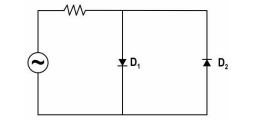(b) Consider a network ABCD containing two identical diodes D1 and D2. The two diodes offer a resistance of 30 Ω when in forward bias and infinite resistance when in reverse bias.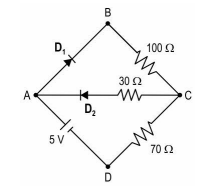If the maximum current that can flow in either of the diodes is 30 mA beyond which they burn out, determine which of the diodes are safe in the above circuit?

7. (a) Given are two nuclei: 1𝐻 3 and 2𝐻𝑒 3 . Justify that these two nuclei have the same radii.

(b) A scientist hypothesizes the existence of a neutral particle of mass 2.0272 u which consists of two neutrons only. Take mass of neutron = 1.0086 u.

i. What is the mass defect of this particle?

ii. Can such a particle exist? Give a reason for your answer

8. A student obtains an interference pattern formed in Young’s double-slit experiment using a yellow light in the physics lab.
(a) Suggest any two methods the student can use to increase the distance between the fringes.
(b) What will happen if the student shifts the source such that it is slightly above the perpendicular bisector of the two slits?
(c) Compare the distance between central and first maxima with that between second and third maxima obtained in the interference pattern.

9. Radiations of different frequencies fall on two different metal surfaces. The graph below represents the kinetic energy of the emitted photoelectrons as a function of frequencies of incident radiations.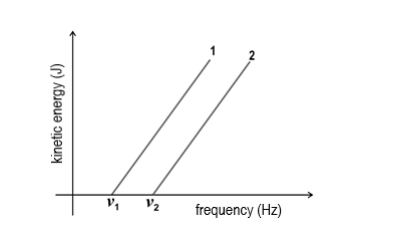(a) If the two metals along with their work functions are: Potassium (2.3 eV) and Aluminium (4.3 eV), identify which line represents each metal. Give a reason for your answer.
(b) Write the photoelectric equation that represents each of the above graphs. Find the slope of the graph.
(c) State one condition with respect to incident radiation for which the photoelectric current produced by it is proportional to its intensity.

10. An object is placed at a distance of 4.5 cm to the left of a convex lens A of focal length 4 cm.
(a) Find the distance from lens A where a screen should be placed to obtain the image.
(b) The screen is removed and the image is used as an object for a diverging lens B of focal length 2 cm so that a real image is formed at a distance of 8 cm from lens B. Find the distance between lens A and lens B.

11. (a) Electromagnetic waves are believed to carry energy and momentum. Give one illustrative example that proves this characteristic of em waves.
(b) What is one common function of each of these? Ozone layer, Glass windows, Special glass goggles used while welding metals
(c) Write an equation that gives the speed of an electromagnetic wave in a material medium in terms of the electric and magnetic properties of the medium. Compare the speed of the em wave in a material medium and in vacuum.
OR
(a) A red light of wavelength λ passes through a single slit of width w and produces a diffraction pattern on the screen. If the red light is replaced by the green light of wavelength 0.75λ, find the slit width required so as to keep the diffraction pattern for green light the same as that of the red light.
(b) Find the wavelength of the light that produces the first minimum at an angle of 30o through a narrow slit of width 0.8 μm.
(c) State the formula for the width of the central maximum of the single slit diffraction pattern. What will be the impact on the width of the central maximum if the whole of the diffraction apparatus is immersed in a liquid of refractive index n? Explain.

SECTION C – Case Study
12. Ria is walking slowly with her head above the water surface in the swimming pool that is about 1.5 m deep. She spots a coin at the bottom of the pool directly below. The coin appears raised to a depth that is less than the actual depth of the pool. The refractive index of water in the pool can be taken as 1.3.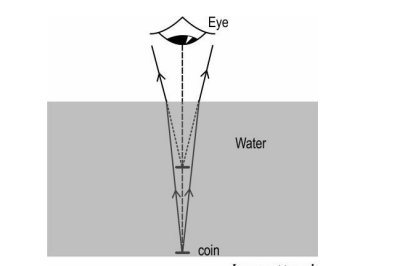Ria then gets under the water and begins looking up at the water surface. A friend of hers holds a coin directly above her eyes in the air. To Ria, the coin appears to be at a height more than the actual height.

In both the above cases, the virtual image of the coin is formed due to the refraction of light at the water-air interface. The bending of the light towards or away from the normal determines the position of the image formed at a distance that is more or less than its real depth/height.

(a) State the formula Ria can use to find the apparent depth of the coin placed at the bottom of the pool.

(b) Find the apparent depth of the coin placed at the bottom of the pool as seen by Ria from the air above the water surface.

(c) If the water in the pool gets polluted with an unknown liquid, thereby decreasing its refractive index, what will be the effect on the apparent depth of the coin placed in water as seen by Ria above the water surface?

(d) Represent the ray diagram for the second case when Ria looks at the coin in the air from inside the water.

(e) Find the apparent height of the coin from the water surface as seen by Ria underwater, when the coin is in the air at a height of 1.5 m from the water surface.

Related Post:

## FAQs on CBSE Class 12 Physics Term 2 Practice Questions 2022

Q. Where I can get CBSE Class 12 Physics Term 2 Practice Questions 2022?

We have given the CBSE Class 12 Physics Term 2 Practice Questions 2022 on this page. The students can get CBSE Class 12 Term 2 Practice Questions 2022 of other subjects from the official website of Adda247 School.

Q. When will CBSE conduct the Class 12 Term 2 Exam of Physics?

The CBSE will conduct the CBSE Class 12 Term 2 Exam of physics on the 20th of May 2022, Friday.

Q. What is the CBSE Class 12 Term 2 Exam Date?

As per the CBSE Class 12 Term 2 date sheet released by the board, the CBSE Class 12 Term 2 Exam 2022 will be held between 26th April to 15th June 2022.

Sharing is caring!

Thank You, Your details have been submitted we will get back to you.